# Metropolis Monte Carlo

The Metropolis Monte Carlo technique  is a variant of the original Monte Carlo method proposed by Nicholas Metropolis and Stanislaw Ulam in 1949 

## Main features

Metropolis Monte Carlo simulations can be carried out in different ensembles. For the case of one-component systems the usual ensembles are:

• Canonical ensemble (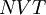$NVT$ )
• Isothermal-isobaric ensemble (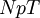$NpT$)
• Grand canonical ensemble (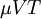$\mu V T$)

In the case of mixtures, it is useful to consider the so-called Semi-grand ensembles. The purpose of these techniques is to sample representative configurations of the system at the corresponding thermodynamic conditions. The sampling techniques make use of the so-called pseudo-random number generators.

## Configuration

A configuration is a microscopic realisation of the thermodynamic state of the system. To define a configuration (denoted as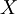$\left. X \right.$ ) we usually require:

• The position coordinates of the particles
• Depending on the problem, other variables like volume, number of particles, etc.

The probability of a given configuration, denoted as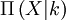$\Pi \left( X | k \right)$, depends on the parameters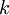$k$ (e.g. temperature, pressure)

Example: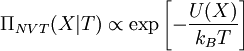$\Pi_{NVT}(X|T) \propto \exp \left[ - \frac{ U (X) }{k_B T} \right]$

In most of the cases$\Pi \left( X | k \right)$ exhibits the following features:

• It is a function of many variables
• Only for a very small fraction of the configurational space the value of$\Pi \left( X | k \right)$ is not negligible.

Due to these properties, Metropolis Monte Carlo requires the use of Importance Sampling techniques

## Importance sampling

Importance sampling is useful to evaluate average values given by: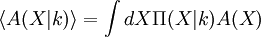$\langle A(X|k) \rangle = \int dX \Pi(X|k) A(X)$

where:

•$\left. X \right.$ represents a set of many variables,
•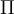$\left. \Pi \right.$ is a probability distribution function which depends on$X$ and on the constraints (parameters)$k$
•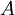$\left. A \right.$ is an observable which depends on the$X$

Depending on the behavior of$\left. \Pi \right.$ we can use to compute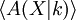$\langle A(X|k) \rangle$ different numerical methods:

• If$\left. \Pi \right.$ is, roughly speaking, quite uniform: Monte Carlo Integration methods can be effective
• If$\left. \Pi \right.$ has significant values only for a small part of the configurational space, Importance sampling could be the appropriate technique

#### Outline of the Method

• Random walk over$\left. X \right.$: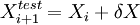$\left. X_{i+1}^{test} = X_{i} + \delta X \right.$

From the configuration at the i-th step one builds up a test configuration by slightly modifying some of the variables$X$

• The test configuration is accepted as the new (i+1)-th configuration with certain criteria (which depends basically on$\Pi$)
• If the test configuration is not accepted as the new configuration then: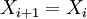$\left. X_{i+1} = X_i \right.$

The procedure is based on the Markov chain formalism, and on the Perron-Frobenius theorem. The acceptance criteria must be chosen to guarantee that after a certain equilibration time a given configuration appears with probability given by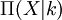$\Pi(X|k)$

## Temperature

The temperature is usually fixed in Metropolis Monte Carlo simulations, since in classical statistics the kinetic degrees of freedom (momenta) can be generally, integrated out. However, it is possible to design procedures to perform Metropolis Monte Carlo simulations in the microcanonical ensemble (NVE).

## Boundary Conditions

The simulation of homogeneous systems is usually carried out using periodic boundary conditions.

## Initial configuration

The usual choices for the initial configuration in fluid simulations are:

• an equilibrated configuration under similar conditions (for example see )
• an ordered lattice structure. For details concerning the construction of such structures see: lattice structures.